# Number Theory 1 Introduction to Number Theory Number

• Slides: 13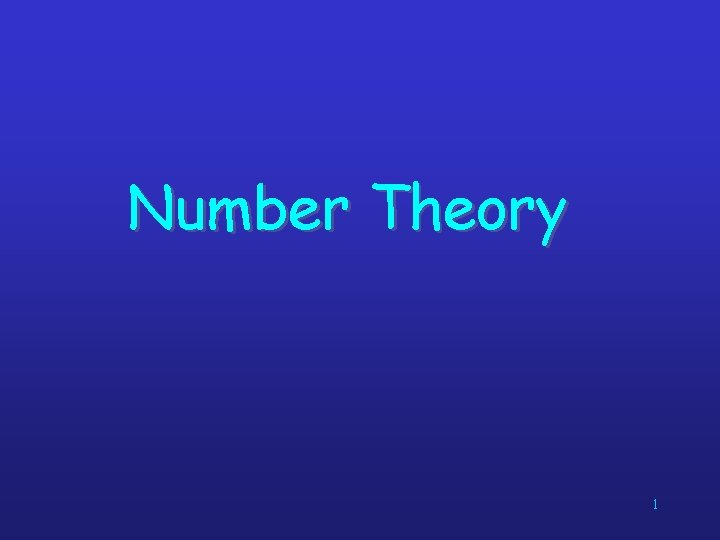Number Theory 1Introduction to Number Theory Number theory is about integers and their properties. We will start with the basic principles of • divisibility, • greatest common divisors, • least common multiples, and • modular arithmetic and look at some relevant algorithms. 2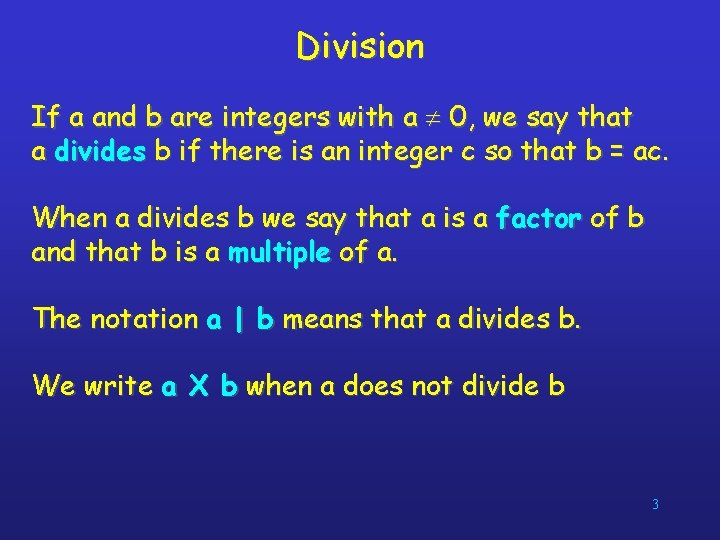Division If a and b are integers with a 0, we say that a divides b if there is an integer c so that b = ac. When a divides b we say that a is a factor of b and that b is a multiple of a. The notation a | b means that a divides b. We write a X b when a does not divide b 3Divisibility Theorems For integers a, b, and c it is true that • if a | b and a | c, then a | (b + c) • if a | b, then a | bc for all integers c • if a | b and b | c, then a | c 4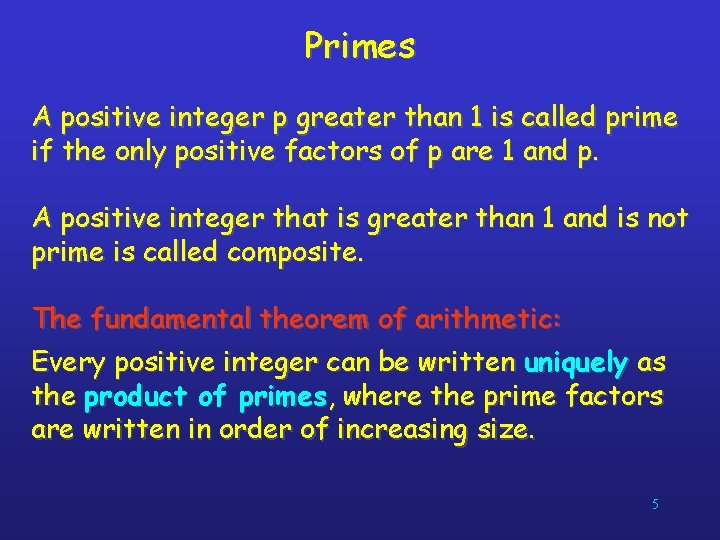Primes A positive integer p greater than 1 is called prime if the only positive factors of p are 1 and p. A positive integer that is greater than 1 and is not prime is called composite. The fundamental theorem of arithmetic: Every positive integer can be written uniquely as the product of primes, where the prime factors are written in order of increasing size. 5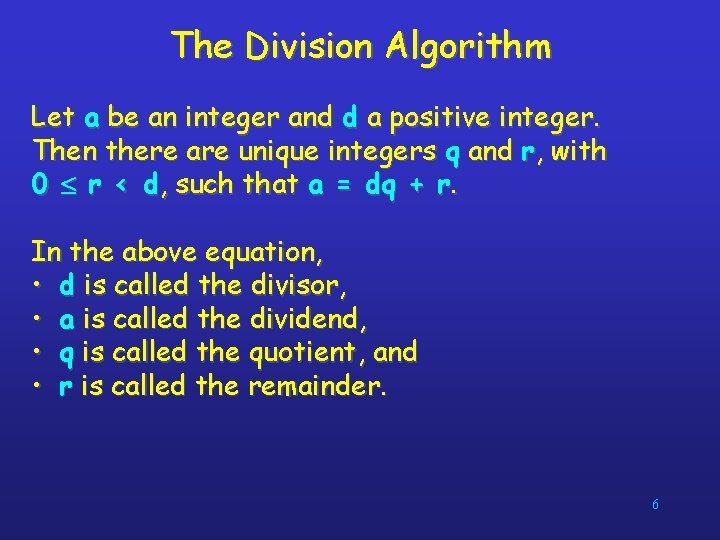The Division Algorithm Let a be an integer and d a positive integer. Then there are unique integers q and r, with 0 r < d, such that a = dq + r. In the above equation, • d is called the divisor, • a is called the dividend, • q is called the quotient, and • r is called the remainder. 6Greatest Common Divisors Let a and b be integers, not both zero. The largest integer d such that d | a and d | b is called the greatest common divisor of a and b. The greatest common divisor of a and b is denoted by gcd(a, b). Example 1: What is gcd(48, 72) ? The positive common divisors of 48 and 72 are 1, 2, 3, 4, 6, 8, 12, 16, and 24, so gcd(48, 72) = 24. Example 2: What is gcd(19, 72) ? The only positive common divisor of 19 and 72 is 1, so gcd(19, 72) = 1. Fall 2002 CMSC 203 - Discrete Structures 7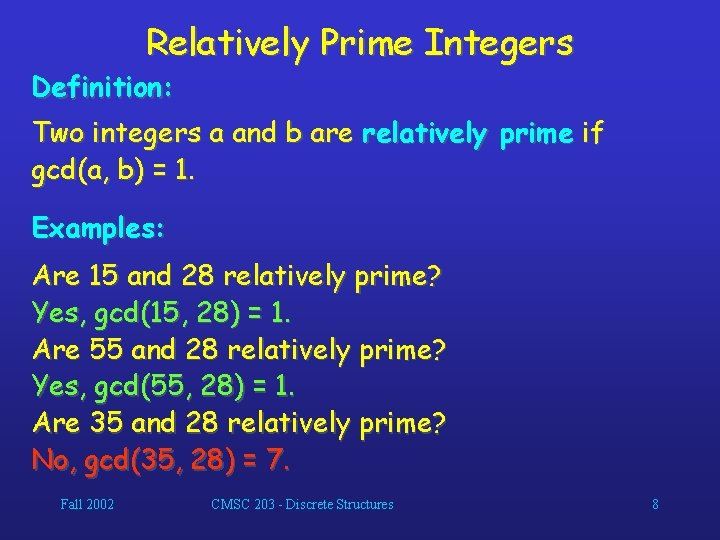Relatively Prime Integers Definition: Two integers a and b are relatively prime if gcd(a, b) = 1. Examples: Are 15 and 28 relatively prime? Yes, gcd(15, 28) = 1. Are 55 and 28 relatively prime? Yes, gcd(55, 28) = 1. Are 35 and 28 relatively prime? No, gcd(35, 28) = 7. Fall 2002 CMSC 203 - Discrete Structures 8Relatively Prime Integers Definition: The integers a 1, a 2, …, an are pairwise relatively prime if gcd(ai, aj) = 1 whenever 1 i < j n. Examples: Are 15, 17, and 27 pairwise relatively prime? No, because gcd(15, 27) = 3. Are 15, 17, and 28 pairwise relatively prime? Yes, because gcd(15, 17) = 1, gcd(15, 28) = 1 and gcd(17, 28) = 1. Fall 2002 CMSC 203 - Discrete Structures 9Least Common Multiples Definition: The least common multiple of the positive integers a and b is the smallest positive integer that is divisible by both a and b. We denote the least common multiple of a and b by lcm(a, b). Examples: lcm(3, 7) = 21 lcm(4, 6) = 12 lcm(5, 10) = 10 Fall 2002 CMSC 203 - Discrete Structures 10Least Common Multiples Using prime factorizations: a = p 1 a 1 p 2 a 2 … p n a n , b = p 1 b 1 p 2 b 2 … p n b n , where p 1 < p 2 < … < pn and ai, bi N for 1 i n lcm(a, b) = p 1 max(a 1, b 1 ) p 2 max(a 2, b 2 ) … pnmax(an, bn ) Example: a = 60 = 22 31 51 b = 54 = 21 33 50 lcm(a, b) = 22 33 51 = 4� 27� 5 = 540 Fall 2002 CMSC 203 - Discrete Structures 11GCD and LCM a = 60 = 22 31 51 b = 54 = 21 33 50 gcd(a, b) = 21 3 1 5 0 =6 lcm(a, b) = 22 3 3 5 1 = 540 Theorem: a�b = gcd(a, b)�lcm(a, b) Fall 2002 CMSC 203 - Discrete Structures 12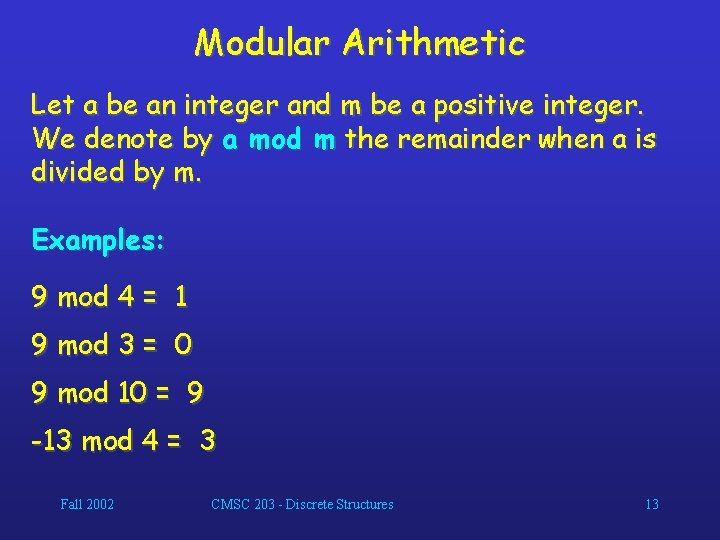Modular Arithmetic Let a be an integer and m be a positive integer. We denote by a mod m the remainder when a is divided by m. Examples: 9 mod 4 = 1 9 mod 3 = 0 9 mod 10 = 9 -13 mod 4 = 3 Fall 2002 CMSC 203 - Discrete Structures 13